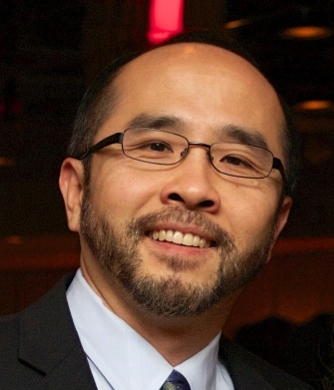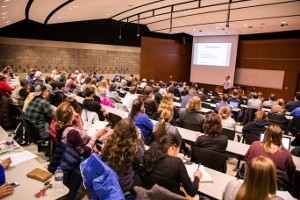# Francis Su delivers the second annual Mosaic LectureFrancis Su, Benediktsson-Karwa Professor of Mathematics at Harvey Mudd College and the President of the Mathematical Association of America, delivered the second annual Mosaic Lecture, titled “My Favorite Math Fun Facts.”  In his talk, he presented a wide range of interesting facts that have been discovered by mathematicians from a diverse collection of countries, cultures, and eras.

Professor Su said that a “math fun fact” can change how we think about mathematics, arouse curiosity in our minds, or even surprise us, while helping us understand that mathematics is more than just numbers or calculus.  It is both science and art:  the science of patterns, and the art of engaging those patterns.

Among the many things the audience learned at the lecture:

- if you pick any number, square it, subtract the original number from the result, divide the latest result by the original number, again subtract the original number from the latest result, and then sequentially add 3 and add 50, the final result will always be the same:  52.  Professor Su impressed us with this example by asking every one of the 100 or so people in attendance to pick their favorite number between 1 and 10, and then mentally follow his algorithm.  At the end, he revealed to us that he knew our final number must be 52.  This “trick” works, of course, because of the power of algebra and that for any nonzero real number x,

(x2 - x) / x - x + 3 + 50 = 52.- every time you shuffle a deck of cards, you're making history: the ordering that results after a deck of cards is shuffled is probably unique in the history of the universe. This is because there are 52! orderings of a deck of cards, which is approximately 1068. This massive number is bigger than the number of stars in the universe (1023), the number of ways to fill out a NCAA bracket (264, which is approximately 1018), and seconds since the Big Bang (1017).

- two different proofs of the Pythagorean Theorem, as well as a version of the Pythagorean Theorem that holds on the unit sphere.  On the unit sphere, it turns out that for a right triangle with arcs of lengths c, a, and (with c opposite the right angle), it follows that

cos(c) = cos(a) cos(b).

Prof. Su then demonstrated how this result holds in a slightly varied form on a non-unit sphere, and how if one lets the radius of the sphere tend to infinity, the planar version of the Pythagorean Theorem emerges.

We also learned that there exists an irrational number r such that rr is a rational number, that sums of cubes of consecutive numbers always produce perfect squares, how to create magic squares, and a card trick that mathematics makes foolproof. Along the way, we met a wide range of historical characters, including Greek and Islamic astronomers, al-Khwarizmi, and President Garfield.

Indeed, as Prof. Su said, “Math is more than numbers or calculus.  Math is both science and art:  the science of patterns, and the art of engaging with those patterns.”  To learn more, see https://www.math.hmc.edu/funfacts/ or download the iPhone app.